+
Standard Normal Distribution
Interpret Inferential Statistics
0
of 0 possible points

# Standard Normal Distribution

Author: Sophia Tutorial
##### Description:

Estimate a z-score given a standard normal distribution and corresponding percentage.

(more)

Sophia’s self-paced online courses are a great way to save time and money as you earn credits eligible for transfer to many different colleges and universities.*

No credit card required

37 Sophia partners guarantee credit transfer.

299 Institutions have accepted or given pre-approval for credit transfer.

* The American Council on Education's College Credit Recommendation Service (ACE Credit®) has evaluated and recommended college credit for 32 of Sophia’s online courses. Many different colleges and universities consider ACE CREDIT recommendations in determining the applicability to their course and degree programs.

Tutorial
what's covered
This lesson discusses standard normal distributions. By the end of this lesson, you will be able to estimate the approximate location of a z-score when given a standard normal distribution. This lesson covers:
1. Standard Normal Distributions
2. Properties of Z-Distributions

## 1. Standard Normal Distributions

Recall from previous lessons that normal distributions have a bell-shaped curve in which the mean is the center point and the standard deviation indicates how spread out the distribution is. One of the goals of using normal distributions is to find the probability under the normal distribution curve that an event will occur.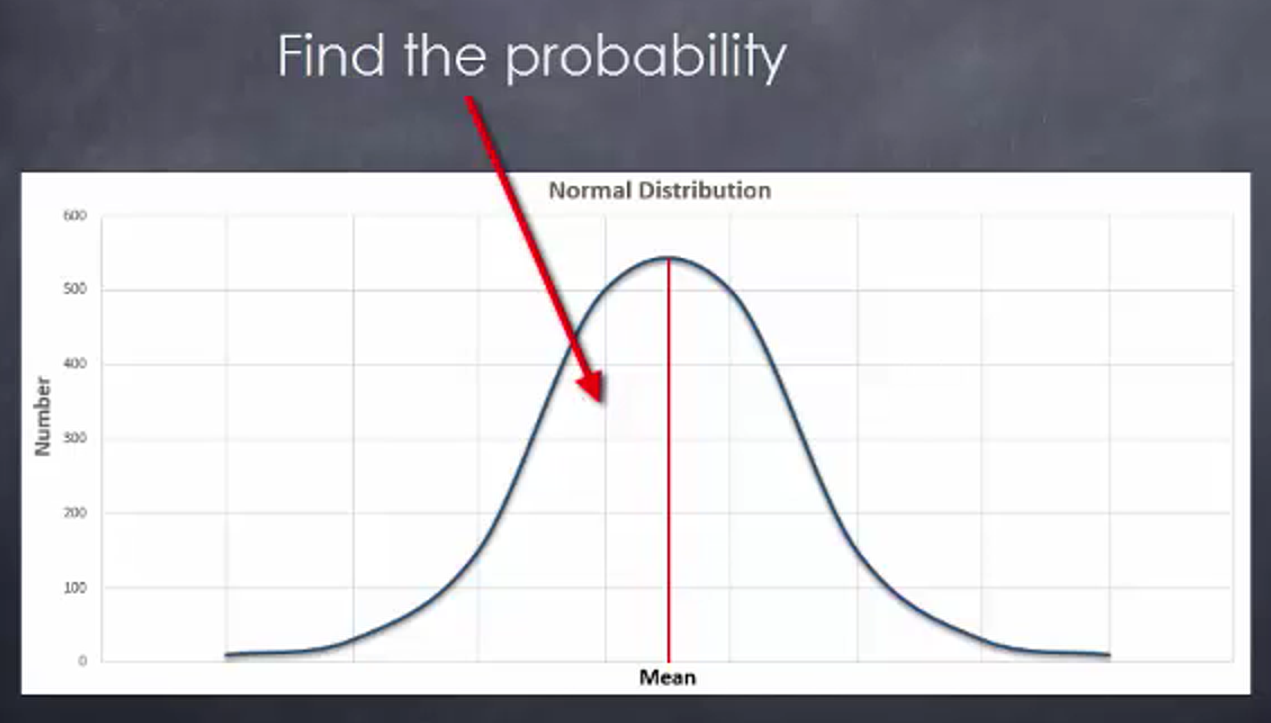Since you are often concerned with finding a probability under the normal distribution curve and because normal distribution curves differ for the data you are considering, there is a need to create a standard normal distribution. This is because doing so will allow you to quickly determine the probabilities related to any normal distribution.

How do you change things from a normal distribution to a standard normal distribution? This is done by simply changing the points of the standard deviations. You do what’s called standardizing them. You can change a normal distribution into a standard distribution by applying a formula to each value in the normal distribution. By converting a normal distribution into a standard normal distribution, each value is changed from the normal distribution into what are referred to as z-scores, by using the following formula:

formula

Z-Score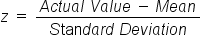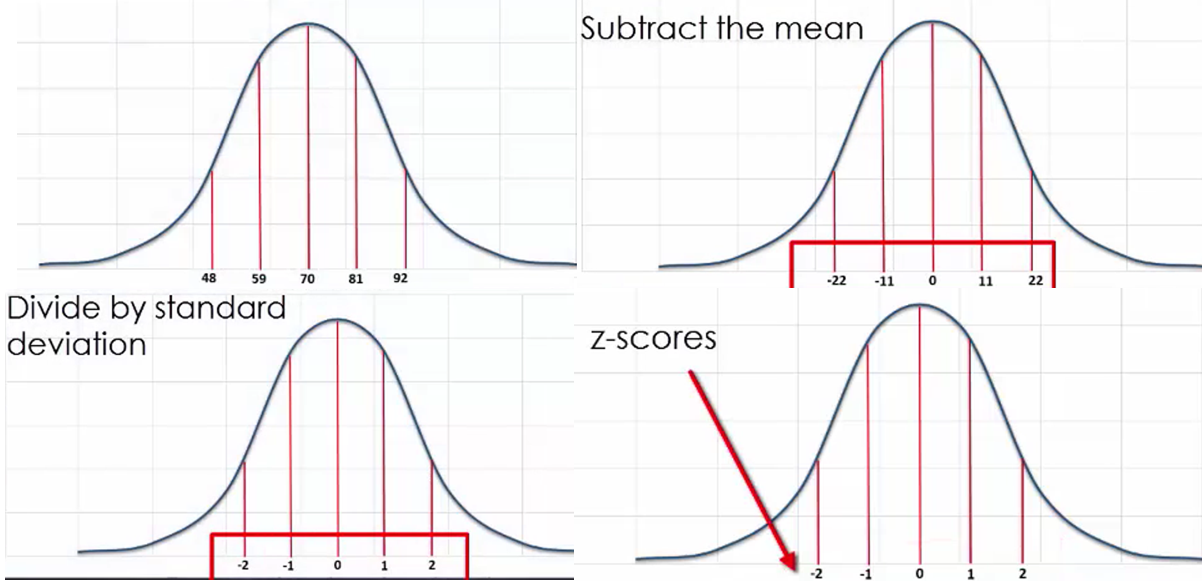## 2. Properties of Z-Distributions

Z-distributions are used to define the position of a value in terms of the mean and the standard deviation where the mean has a z-score equal to 0 due to its being a location along the horizontal axis. This axis is the one that provides us with the location of any z-score.

What is interesting about a z-distribution is that its shape contains what are called “turning points.” These fall on the curve at the point where a z-score is equal to either -1 or 1. The left turning point has a z-score of -1, which indicates its location on the horizontal axis, whereas the right turning point has a z-score of 1 since that is where it is located on the horizontal axis.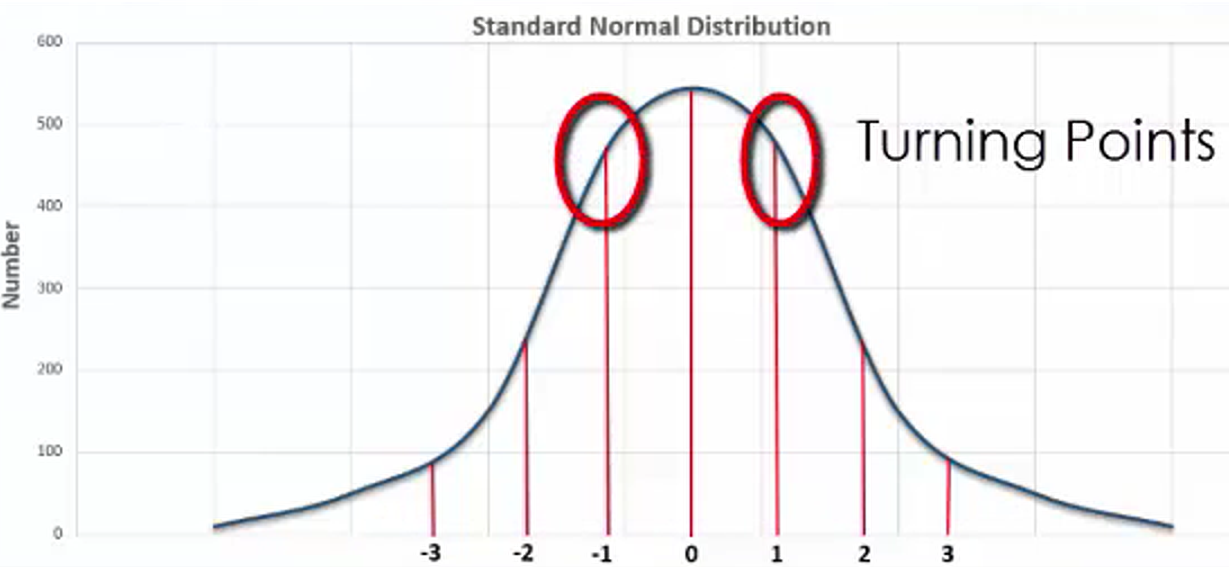Additionally, there are typically values of -3, -2, 2, and 3 on the z-distribution. What is especially useful about the z-distribution graph is that the areas between different z-scores are known, which makes it relatively easy to determine the probability that a specific event will occur.

Roughly 68% of the area under the z-distribution curve falls between the z-score values of -1 and 1.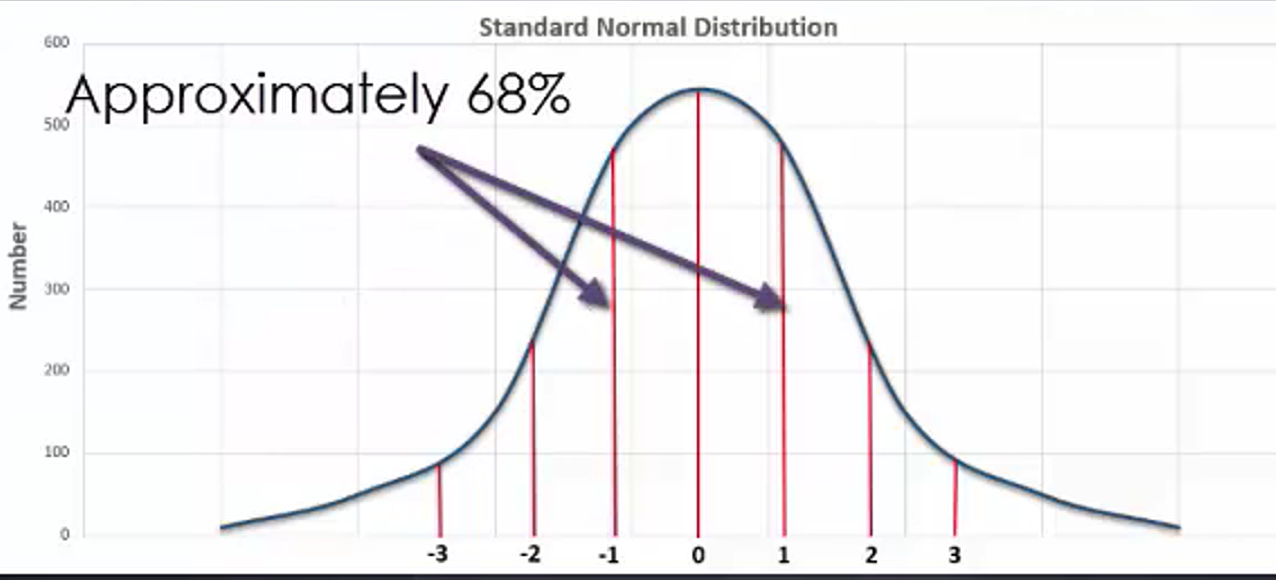In addition, approximately 95% of the area under the z-distribution curve falls between the z-scores of -2 and 2.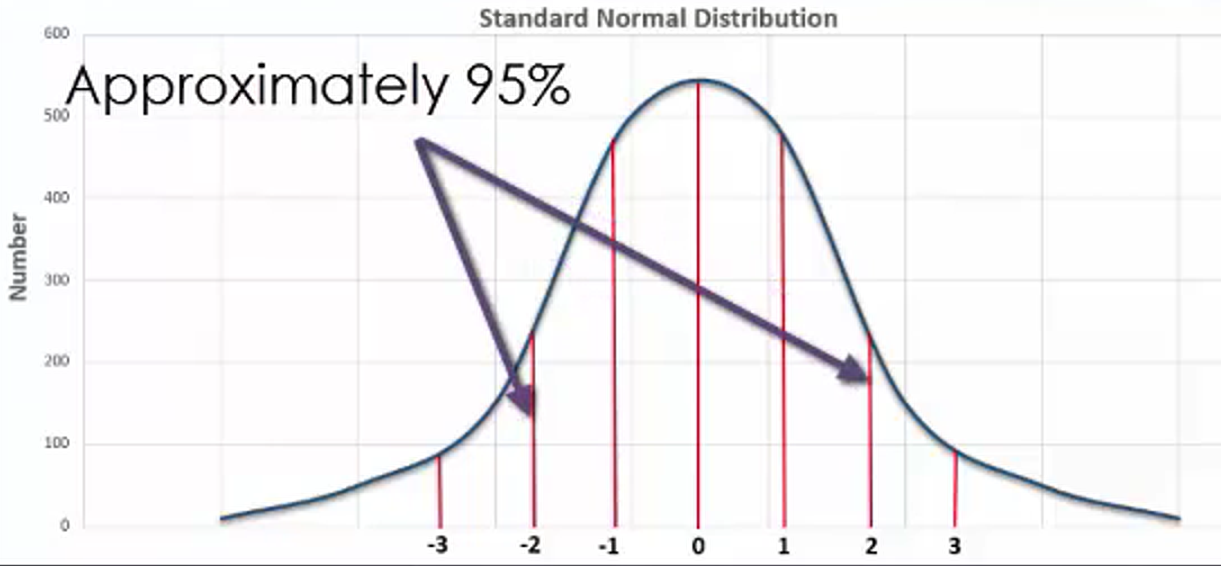Approximately 99.7% of the area under the z-distribution curve falls between the z-score values of -3 and 3.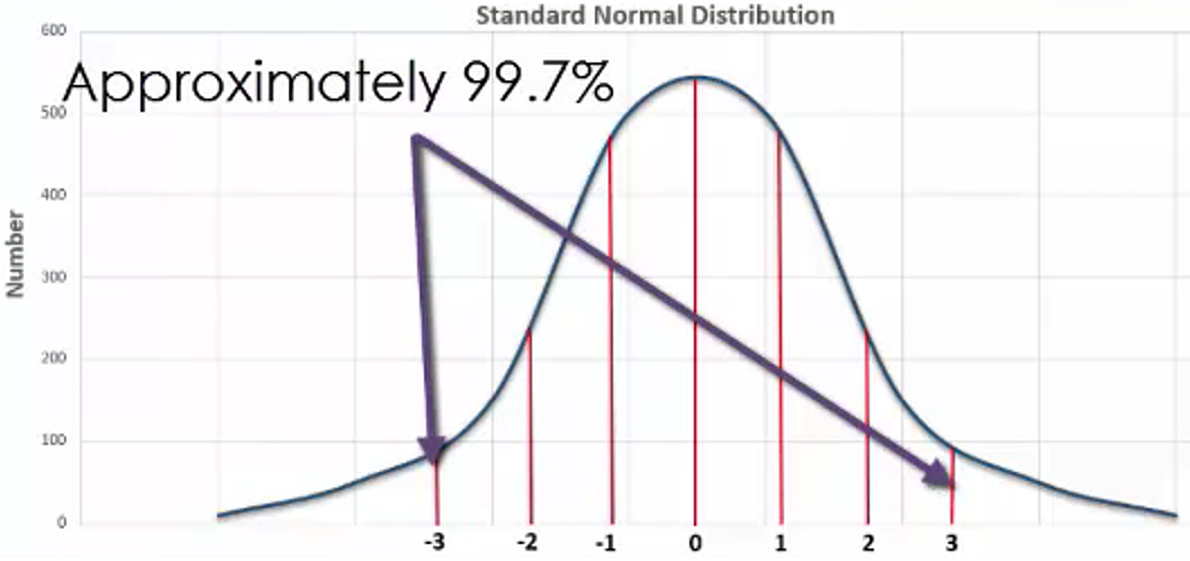A z-score less than -3 and greater than 3 account for the remaining 0.3% of the area under the z-distribution curve.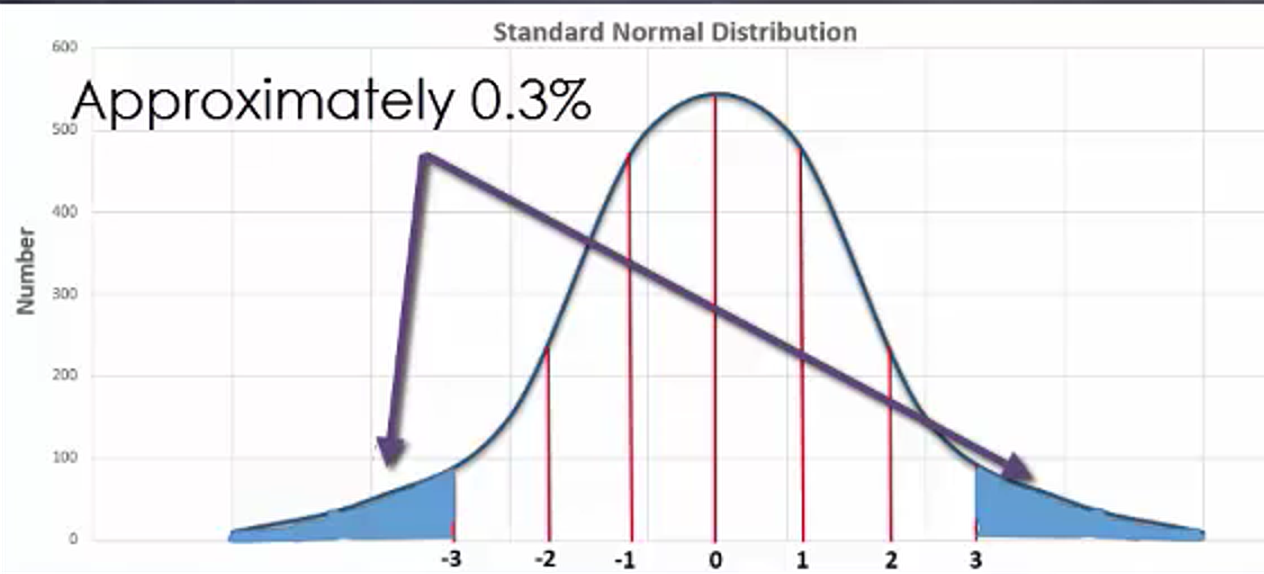Each one of these percentage values is related to a probability value that explains the chance of an event occurring if it happens to fall between two specific z-scores.

big idea
The mean is located on the point where z is equal to 0 on the horizontal axis of a z-distribution. There are also points on this distribution to indicate where the z-scores of -3, -2, -1, 1, 2, and 3 are located. When considering the area under the curve that falls between a z-score of -1 and 1, the proportion of this area is approximately 68% of the total area under the curve. The area between z = -2 and z = 2 represents approximately 95% of the area under the curve of the whole z-distribution.
What does it mean if you have z = 0.75 and z = 1.72?

These are values that are likely to occur, as they fall within the middle 95% of the distribution. However, z-scores such as -3.14 and 4.87 fall somewhat far from the mean score of z = 0. Therefore, these values are quite unlikely to occur.
summary
This lesson showed how to estimate the approximate location of a z-score when provided with a standard normal distribution. You can do this because of the properties of z-distribution. The scores reflect known values, which can be used to determine probability.

Source: This work is adapted from Sophia author Dan Laub.

Formulas to Know
Z-ScoreRating